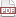### Raking

19 November 12. [link]PDF version

It's called iterative proportional fitting (IPF), but why use a three-letter acronym (TLA) when you have a descriptive name?

The problem starts with one table, and a set of sums along the margins:

 2 3 5 8 7 15 10 10

We like these margins and feel them to be relevant. For example, they may be the results of a carefully-run census.

Now we have another table, say a quick-and-dirty survey that we want to reweight to the census.

 7 9 16 12 7 19 19 16

It has its own marginal totals, but we're overriding them to match the margins of the census: row 1 will have sum 5, row 2 will sum to 15, and the columns will each sum to ten.

Our 2×2 table has four degrees of freedom (three if we want to reweight the table to sum to one, as is sometimes done) and the row and column restrictions cover two degrees, leaving us either two or one degree of freedom along which to adjust the survey table.

So there's the problem setup: we want to adjust the survey results to the closest table that conforms to the reference margins.

This is a pretty versatile setup. In the next few episodes, we'll use it to generate synthetic data, impute missing data, adjust unconstrained data to cell-by-cell constraints, run an OLS regression on categorical inputs, and who knows what else.

I think of raking as a method to find the closest point in the space of tables that matches a given constraint. This is distinct from the historical development of the method, about finding the solution of log-linear models, which were all the rage in the 1940s, but largely lost fashion relative to OLS techniques (which are not equivalent, though we'll replicate one regression with a raking later). Regardless of the history, thinking about the approach as a constrained optimization makes much more sense (to me at least), so I will focus on that.

So raking is a constrained optimization, and there are many techniques avaialable that would work to solve the 1- or 2-df problem above. At work, I had the problem of doing this on a table with more dimensions: 60,164 blocks × 2 sexes × 103 ages × 7 races × 2 ethnicities × 11 relationships to the head of the household = 1,908,642,736 cells. However you count it, that's pushing two billion degrees of freedom, and a two-billion-dimensional constrained optimization is not something we can use any old method on.

The method of raking is easy to visualize. Given the 2×2 table above, we'd first re-scale the columns so that each sums to the desired column total. Then, we re-scale the rows so that each sums to the desired row total. Of course, the by-row rescaling throws off the column totals, so we re-scale the column totals. Repeat, raking columns then rows, columns then rows, and you will eventually converge to the table that is closest (by K-L divergence) to the original table, and where the row/column totals are arbitrarily close to those sought. This is a blog, so I don't have to prove anything, but the method was first presented by Deming and Stephan (1940).

Also, that two-billion cell table was sparse, with only 2,599,615 nonempty cells. Apophenia uses that sparseness to make solving this a doable task.

###### An example
Lately, I've been a fan of writing shell scripts for my demos. This one will write two data files to a database, produce a makefile and a short C program, then compile and run the program. You'll need to have installed a recent version of Apophenia (and its dependencies) to make this work; given that you can cut/paste it onto the command line.

apop_text_to_db -O  -d="|" '-' margins sample.db <<"----------"
row | col | weight
1 |  1 |   2.5
1 |  2 |   2.5
2 |  1 |   7.5
2 |  2 |   7.5
----------

apop_text_to_db -O  -d="|" '-' sample sample.db <<"----------"
row | col | val
1 |  1 |   7
1 |  2 |   9
2 |  1 |   12
2 |  2 |   7
----------

cat <<"----------" > rake.c
#include <apop.h>

int main(){
apop_db_open("sample.db");
apop_data_show(
apop_rake(.margin_table="margins", .count_col="weight",
.contrasts=(char*[]){"row", "col"}, .contrast_ct=2,
.init_table="sample", .init_count_col="val",)
);
}
----------

export CFLAGS="-g -Wall -O3  pkg-config --cflags apophenia"
export LDLIBS="pkg-config --libs apophenia"
export CC=c99
make rake

./rake


For the lazy, here's the output.

 row col Weights 1 1 1.77567 1 2 3.22433 2 1 8.22433 2 2 6.77567

You can also try different starting points in the survey table and see how they behave differently in the outcomes.

Next time: raking for missing data. Also, did you notice that the data for the margins in the example add up to the margins as above, but are a bit more even than the original data? That will be useful for synthetic data, which I'll cover as a trivial extension in two episodes.